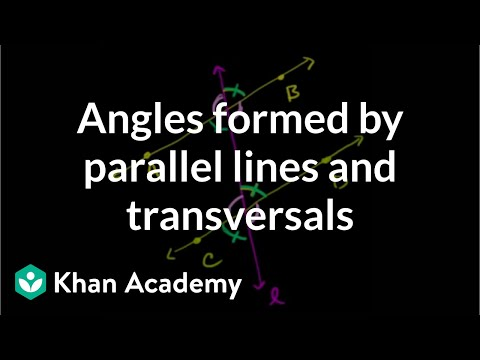Video

# Worked example: Triangle angles (intersecting lines) (Full video)

Description: Worked examples finding angles in triangles formed by intersecting lines. Created by Sal Khan. We're given a bunch of lines here that intersect in all different ways and form triangles. And what we want to do in this video is figure out what the measure of this angle is. Let's see, that's the same thing as 80 minus 21.

### Other videos you might be interested in### Angles in a triangle sum to 180° proof (Full video)